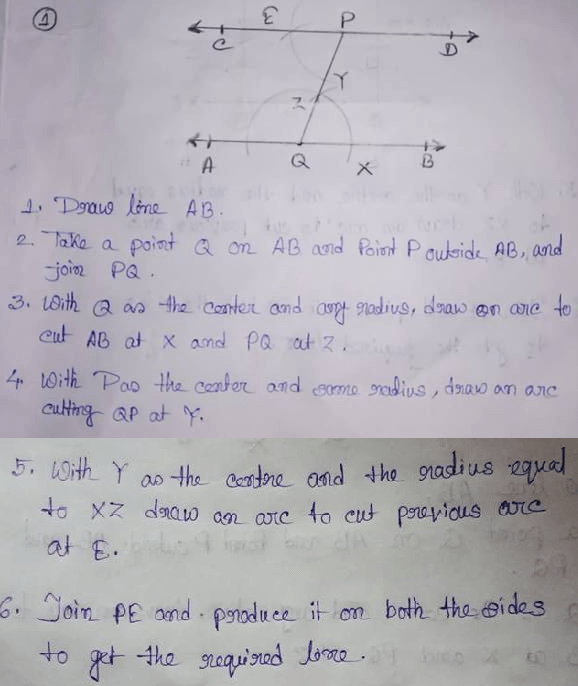# RS Aggarwal Class 7 Math Seventeenth Chapter Constructions Exercise 17A Solution

## EXERCISE 17A

(1) Draw a line AB and take a point P outside it. Draw a line CD parallel to AB and passing through the point P.(2) Draw a line AB and draw another line CD parallel to AB at a distance of 3.5 cm from it.(i) Let AB be the given line.

(ii) Take any two points PQ and Q on AB.

(iii) Construct ∠BPE = 90o and ∠BQF = 90o.

(iv) With P as centre and radius equal to 3.5 cm, cut PE at R.

(v) With Q as centre and radius equal 3.5 cm, cut QF at S.

(vi) Join CD and produce it on either side to get the required line parallel to AB at a distance of 3.5 cm from it.

(3) Draw a line l and draw another line m parallel to l at a distance of 4.3 cm from it.(i) Let l be the given line.

(ii) Take any two points A and B on l.

(iii) Construct ∠BAE = 90o and ∠QBF = 90o.

(iv) With A as centre and radius equal to 4.3 cm, cut AE at C.

(v) With B as centre and radius equal 4.3 cm, cut BF at D.

(vi) Join CD and produce it on either side to get the required line m, parallel to l at a distance of 4.3 cm from it.

For more exercise solutions, Click Below –

Updated: May 30, 2022 — 1:38 pm